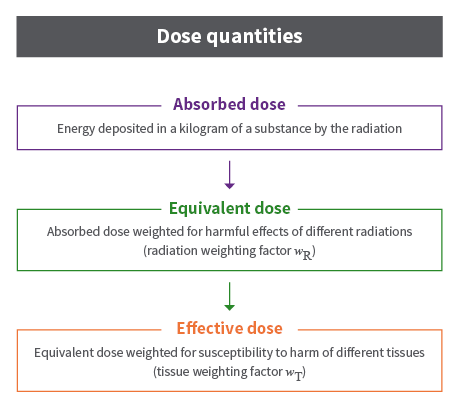# Units of ionising radiation measurement

## Units

Australia uses the International system (SI) of units. The USA uses a different system which can lead to confusion. The following explanation focuses on SI units.

We measure two general phenomena when we discuss radiation. We measure "activity" and "exposure". Activity is basically just how much radiation is coming out of something, whether it's particles or waves. Exposure measures the effect of radiation on substances that absorb it.

Radiation activity is measured in an international (SI) unit called a becquerel (Bq). The becquerel counts how many particles or photons (in the case of wave radiation) are emitted per second by a source. The device used for measurement is often the familiar Geiger counter. If you put a Geiger counter over a gram of substance and count 3 clicks per second, the radioactivity of that substance would be 3 becquerel.

Radiation exposure is expressed in several ways to account for the different levels of harm caused by different forms of radiation and the different sensitivity of body tissues.## Absorbed Dose

Radiation exposure is measured in an international (SI) unit called the gray (Gy). The radiation exposure is equivalent to the energy "deposited" in a kilogram of a substance by the radiation. Exposure is also referred to as absorbed dose. The important concept is that exposure is measured by what radiation does to substances, not anything particular about the radiation itself. This allows us to unify the measurement of different types of radiation (i.e., particles and wave) by measuring what they do to materials.

The gray is a large unit and for normal radiation protection levels a series of prefixes are used:

• nanogray (nGy) is one thousand millionth of a gray (1/1,000,000,000)
• microgray (µGy) is one millionth of a gray (1/1,000,000)
• milligray (mGy) is one thousandth of a gray (1/1,000)

## Equivalent Dose

Often we are interested in the effect of radiation exposure on human tissue. Enter a quantity called equivalent dose. This relates the absorbed dose in human tissue to the effective biological damage of the radiation. Not all radiation has the same biological effect, even for the same amount of absorbed dose. Equivalent dose is measured in an international (SI) unit called the sievert (Sv). Like the gray, the sievert is a large unit and for normal radiation protection levels a series of prefixes are used:

• nanosievert (nSv) is one thousand millionth of a sievert (1/1,000,000,000)
• microsievert (µSv) is one millionth of a sievert (1/1,000,000)
• millisievert (mSv) is one thousandth of a sievert (1/1,000)

To determine equivalent dose (Sv), you multiply absorbed dose (Gy) by a radiation weighting factor that is unique to the type of radiation. The radiation weighting factor (WR) takes into account that some kinds of radiation are inherently more dangerous to biological tissue, even if their "energy deposition" levels are the same.

For x-rays and gamma rays and electrons absorbed by human tissue, WR is 1. For alpha particles it is 20. To compute sieverts from grays, simply multiply by WR. This is obviously a simplification. The radiation weighting factor WR approximates what otherwise would be very complicated computations. The values for WR change periodically as new research refines the approximations.

## Effective Dose

The probability of a harmful effect from radiation exposure depends on what part or parts of the body are exposed. Some organs are more sensitive to radiation than others. A tissue weighting factor (WT) is used to take this into account. When an equivalent dose to an organ is multiplied by the tissue weighting factor for that organ the result is the effective dose to that organ. The unit of effective dose is the sievert (Sv).

If more than one organ is exposed then the effective dose, E, is the sum of the effective doses to all exposed organs.

Tissue Tissue weighting factor
WT
Sum of Tissue weighting factors
Bone-marrow (red), colon, lung, stomach, breast, remaining tissues(*) 0.12 0.72